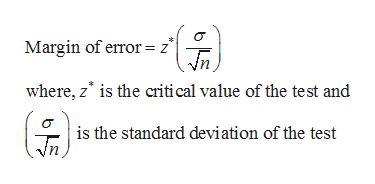# If the sample size is increased and the standard deviation and confidence level stay the same, then the margin of error will also be increased.TrueFalse

Question
212 views

If the sample size is increased and the standard deviation and confidence level stay the same, then the margin of error will also be increased.

• True
• False
check_circle

Step 1

Margin of error:

The margin of error helps in constructing confidence intervals, which gives a range of plausible values for the population parameter. Margin of error is nothing but a range of values above and below the value of sample statistic.

For example, the formula of margin of error for sample mean of z-test is given below.help_outlineImage TranscriptioncloseMargin of error z° where, z is the critical value of the test and is the standard deviation of the test fullscreen
Step 2

Properties of Margin of error:

Some of the properties of margin of error is as follows:

• The margin of error value gets smaller as n (sample size) tends to infinity.
• Higher the value of level of significance, higher the margin of error.
• Higher the standard...

### Want to see the full answer?

See Solution

#### Want to see this answer and more?

Solutions are written by subject experts who are available 24/7. Questions are typically answered within 1 hour.*

See Solution
*Response times may vary by subject and question.
Tagged in

### Other